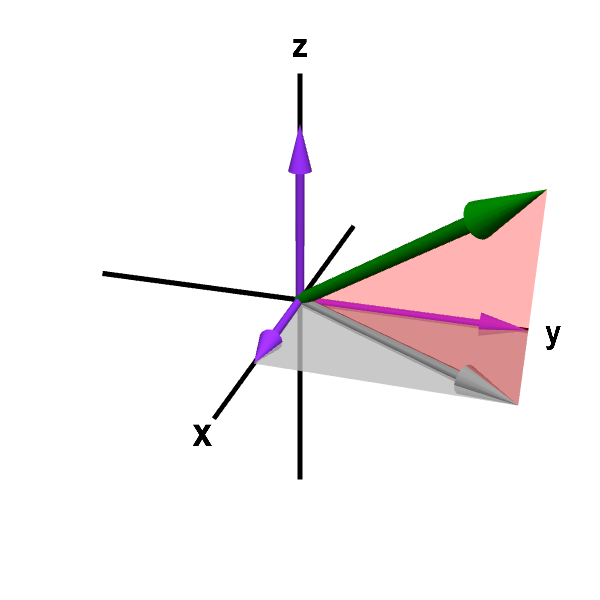# Math Insight

### Applet: A vector in three-dimensional spaceA representation of a vector $\vc{a}=(a_1,a_2,a_3)$ in the three-dimensional Cartesian coordinate system. The vector $\vc{a}$ is drawn as a green arrow with tail fixed at the origin. You can drag the head of the green arrow with your mouse to change the vector. To help show the three dimensional perspective, a pink triangle connects the vector to its projection $(a_1,a_2,0)$ in the $xy$-plane (gray arrow). Purple vectors show the projections of $\vc{a}$ on each axis and represent the coordinates $a_1$, $a_2$, and $a_3$. You can also drag the heads of the purple vectors to change just one of the coordinates of the vector. Or drag the head of the gray vector in the $xy$-plane to change just the $x$ and $y$ coordinates.